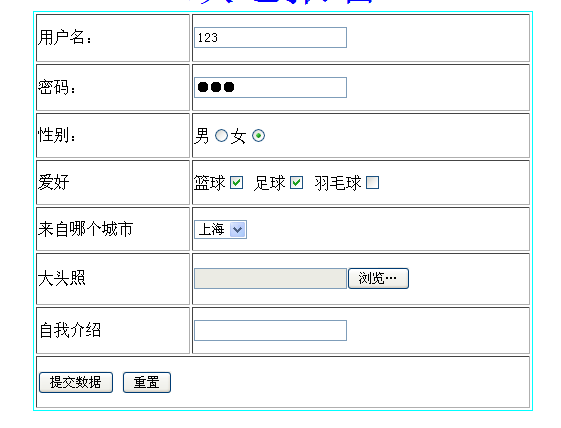# 创建一个HTML的form表格表单<!DOCTYPE html>
<html>
<head>
<meta charset="utf-8" />
<title>用户信息</title>
</head>

<body>

<!--
form表单部分：用来传输数据 是一个整个组件

form属性
action:将表单内容提交的地址
我将提交地址设为了百度_(:зゝ∠)_

method:提交的方式有两种get和post
这里我使用的get方式

-->
<form action="https://www.baidu.com" method="get">

<!--
border用来规定表格边框的宽度
align表格在网页中的对齐方式
cellpadding规定单元边缘和其中内容的空白大小
cellspacing规定单元格之间的空白大小
-->
<table border="1" align="center" cellpadding="1" cellspacing="2" >
<tr>
<td width="125" height="35">用户名：</td>
<td width="300" ><input type="text" name="username"/></td>
</tr>
<tr>
<td width="125" height="35">密码：</td>
<td width="300"><input type="password" name="pwd"/></td>
</tr>
<tr>
<td width="125" height="35">性别:</td>
<td width="300">
<input type="radio" name="sex" value=1 />男
<input type="radio" name="sex" value=0 />女
</td>
</tr>
<tr>
<td width="125" height="35">爱好：</td>
<td width="300">
<input type="checkbox" name="hobby" value="basketball" />篮球
<input type="checkbox" name="hobby" value="football" />足球
<input type="checkbox" name="hobby" value="badminton" />羽毛球
</td>
</tr>
<tr>
<td width="125" height="35">来自：</td>
<td width="300">
<select>
<option value="上海">上海</option>
<option value="北京">北京</option>
<option value="广州">广州</option>
<option value="成都">成都</option>
<option value="郑州">郑州</option>
</select>
</td>
</tr>
<tr>
<td width="125" height="35">照片：</td>
<td width="300">
<input type="file" name="photo"
</td>
</tr>
<tr>
<td width="125" height="35">介绍：</td>
<td width="300">
<textarea cols="50" rows="5"></textarea>
</td>
</tr>
<tr>
<td width="125" height="35" colspan="2">
<input type="submit" value="提交数据"/>
<input type="reset" />
</td>

</tr>
</table>
</form>
</body>
</html>


<table> 标签定义 HTML 表格。

<tr> 标签定义 HTML 表格中的行。
tr 元素包含一个或多个 th 或 td 元素。

<td> 标签定义 HTML 表格中的标准单元格。
HTML 表格有两类单元格：

td 元素中的文本一般显示为正常字体且左对齐。